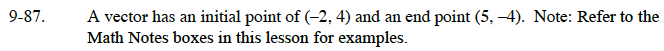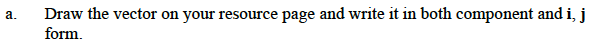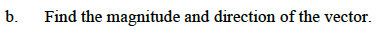### Home > CALC > Chapter 9 > Lesson 9.3.1 > Problem9-87

9-87.
1. A vector has an initial point of (−2, 4) and an end point (5, −4). Note: Refer to the Math Notes boxes in this lesson for examples. Homework Help ✎

1. Draw the vector on your resource page and write it in both component and i, j form.

2. Find the magnitude and direction of the vector.$\text{magnitude}=\sqrt{(x_2-x_1)^2+(y_2-y_1)^2}$

$\text{direction}=\tan^{-1}\Big(\frac{y_2-y_1}{x_2-x_1}\Big)$